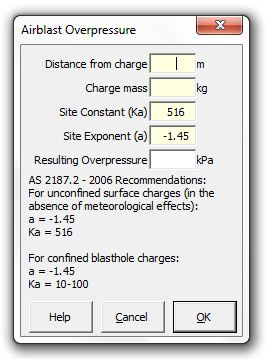### Strutt Help

Airblast Overpressure   1/1, 1/3

Strutt|Vibration|Blasting|Airblast calculates the estimated airblast overpressure given the distance from the explosive charge (m) and the mass of the explosive charge (kg) and inserts the result into the active row.Strutt implements the formula as:

P = K_a*(r/Q^(1//3))^a

Where:
P is the calculated pressure, kPa
Q is the mass of the explosive charge, kg
r is the distance from the explosive charge, m
K_a is the site constant
a is the site exponent

AS2187.2-2006 recommends using a site exponent a of -1.45 and a site constant K_a of 516 for unconfined surface charges, in situations that are not affected by meteorological conditions.

For confined blasthole charges, when using a site exponent a of -1.45, the site constant K_a is commonly in the range 10 to 100.

Reference:

• AS2187.2-2006